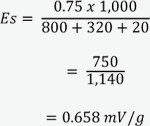Home/Resources/Ask The Experts/Voltage output from a charge-mode piezoelectric accelerometer

# Voltage output from a charge-mode piezoelectric accelerometer

### Question

I have an Endevco® model 2225 accelerometer and I want to perform a one-time drop test. I cannot justify the purchase of a charge amplifier at this time. It there a way I can connect the accelerometer directly to my digital oscilloscope and obtain meaningful data?

While piezoelectric accelerometers are specified with a charge sensitivity, a voltage is also produced. Datasheets from very old accelerometers specify a voltage output because in early days, the charge amplifier had not yet been developed.

When using a charge amplifier, the user need not be concerned about calibration as a function of cable length. The typical oscilloscope has a voltage amplifier input, so we must work with the available voltage from the accelerometer.

The relationship between voltage and charge is best expressed by the equation: E= Q/C

Where:

• E = Voltage

• Q = Charge

C = Capacitance

In actual practice the term C must not only take into account the accelerometer's capacitance, but also external capacitance such as cable length. Capacitance will increase as the length of the cable so it is necessary to know the cable capacitance. Keep in mind that if the length of the cable is increased, the sensitivity will drop accordingly.

A practical equation to determine the sensitivity in mV/g is: Es = (1000 Qs)/(Cp+Ct)

• Es = voltage sensitivity, mV/g

• Qs = charge sensitivity, pC/g

• Cp = transducer (accelerometer) capacitance, pF

Ct = total external capacitance pF

Examples of external capacitance include the cable (major contributor) and the input shunt capacitance of the oscilloscope, DAQ, etc. The cable should be low noise piezoelectric cable, which usually has a nominal capacitance of around 30 pF/ft. The input capacitance should come from the applicable datasheet, which is usually specified as X Ohms in parallel with X pF. Twenty pF is a very typical value.

The input resistance, of the DAQ, will also influence the low frequency amplitude accuracy. It is recommended that the resistance be at least 10 megohms. A lower resistance will result in a reduced low frequency response. Using the equation for time constant T = RC, one can determine the approximate low frequency roll off point, where C = total capacitance and R is the input resistance of the measuring device.

As an example, the Endevco® model has a typical charge sensitivity of 0.75 pC/g. We are going to use a 10 foot 3090C cable which has a typical capacitance of 320 pF (32 pF/foot) and we will also include 20 pF as the input capacitance of the DAQ.To determine the minimum frequency, assume an input resistance of 10, megohms times the